CBSE Class 10 Sample Paper for 2020 Boards - Maths Standard

Class 10
Solutions of Sample Papers for Class 10 Boards

## ‘Skysails’ is that genre of engineering science that uses extensive utilization of wind energy to move a vessel in the sea water. The ‘Skysails’ technology allows the towing kite to gain a height of anything between 100 metres – 300 metres. The sailing kite is made in such a way that it can be raised to its proper elevation and then brought back with the help of a ‘telescopic mast’ that enables the kite to be raised properly and effectively. Based on the following figure related to sky sailing, answer the questions: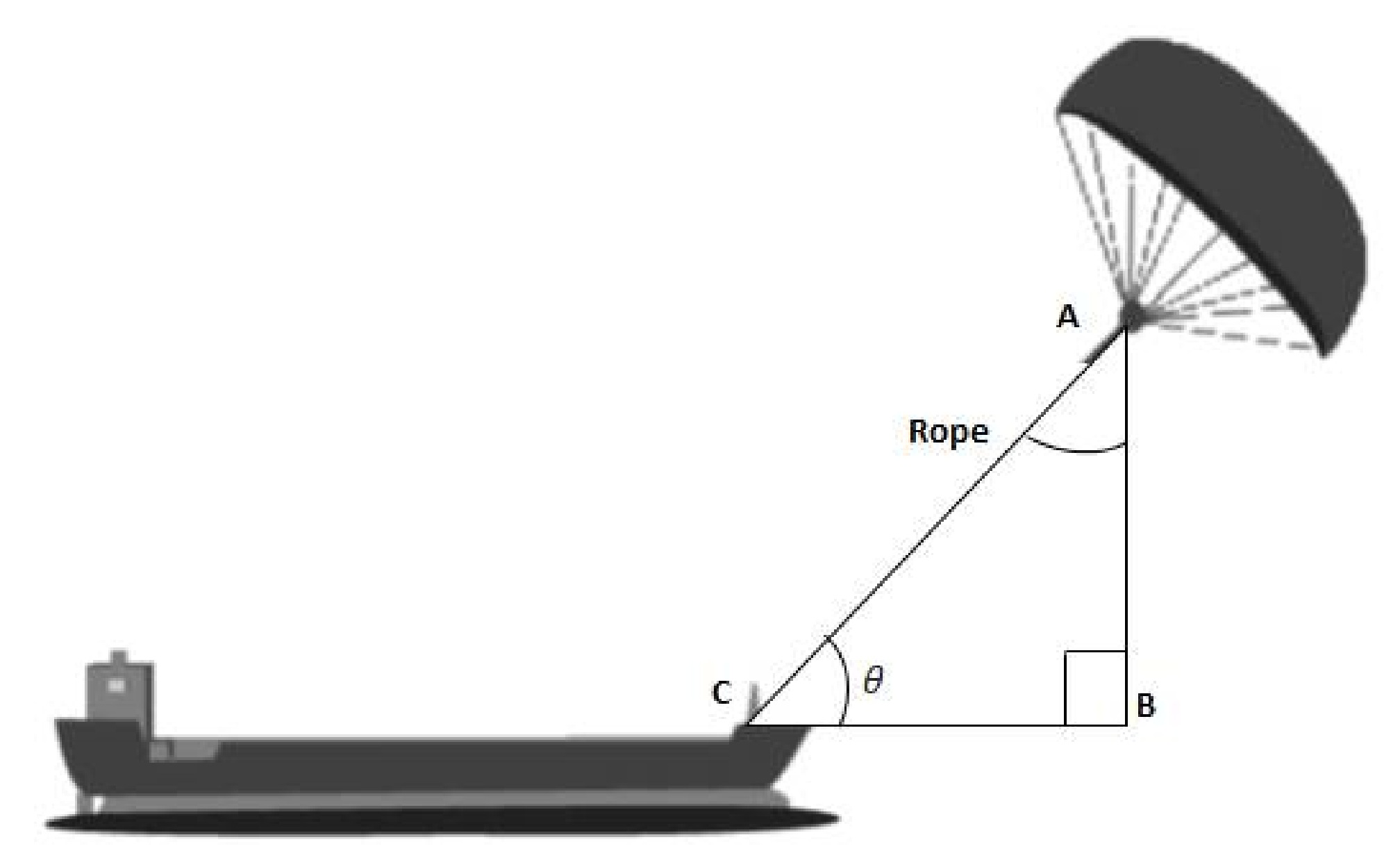## (ii) What should be the length of the rope of the kite sail in order to pull the ship at the angle 𝜃 (calculated above) and be at a vertical height of 200 m?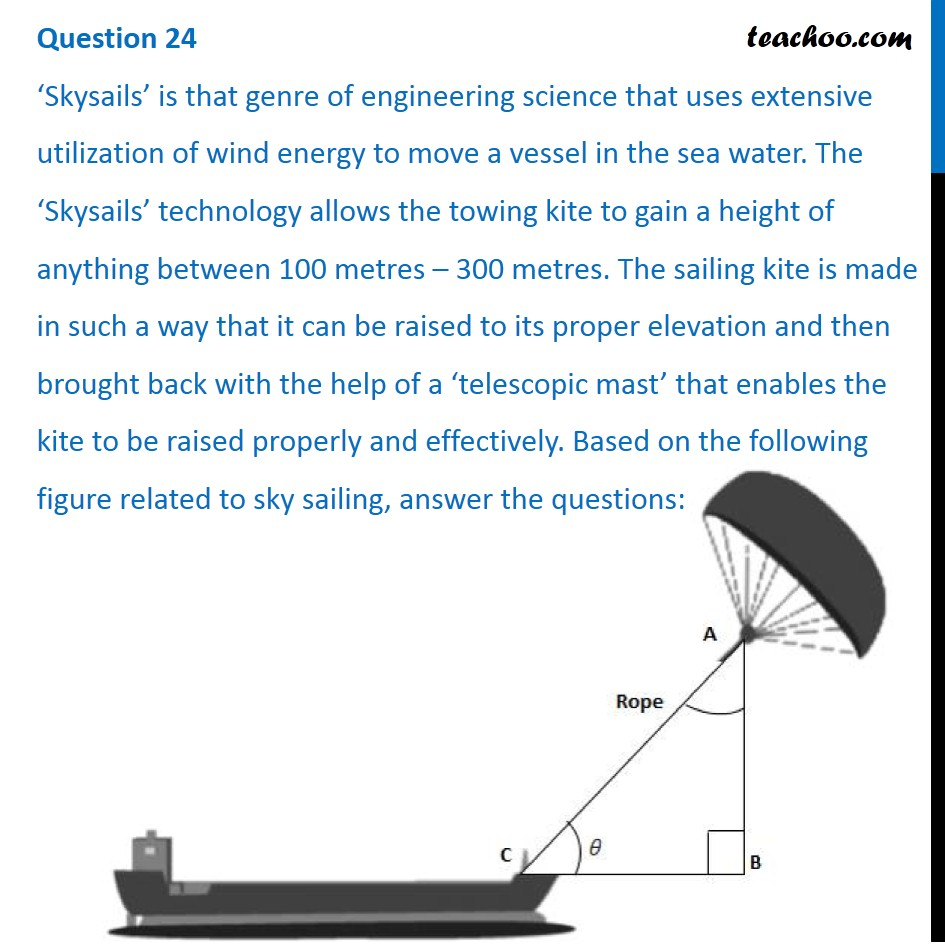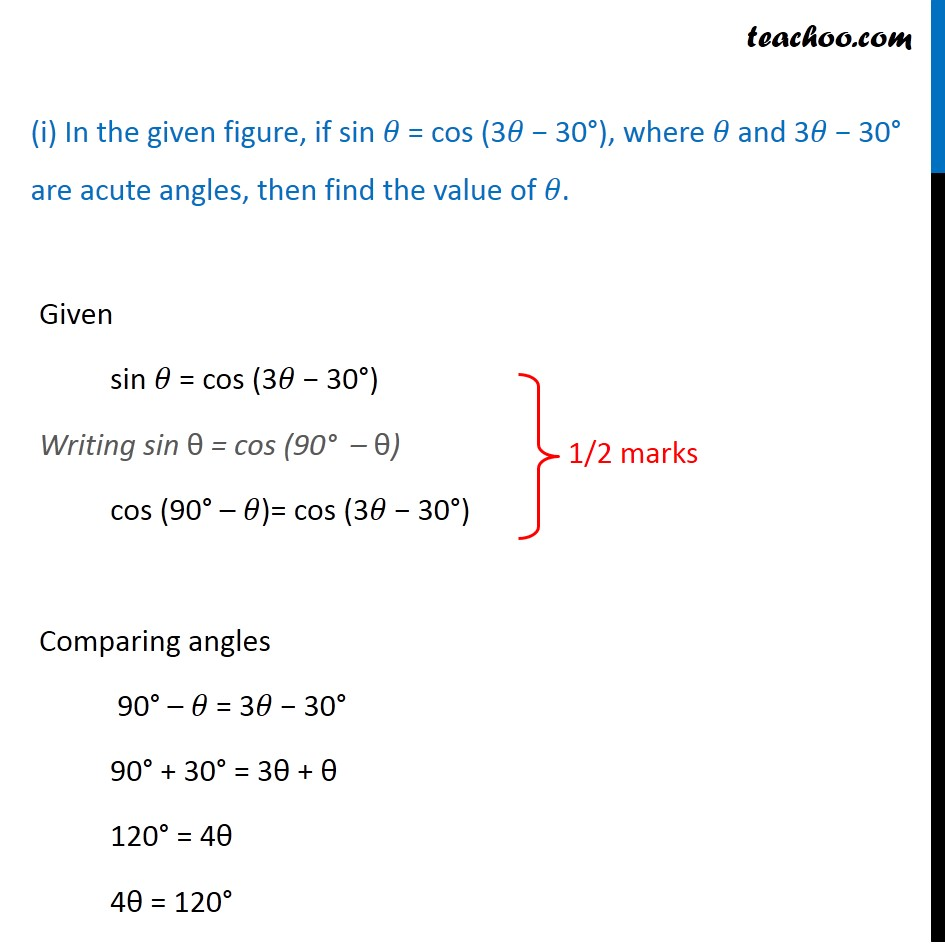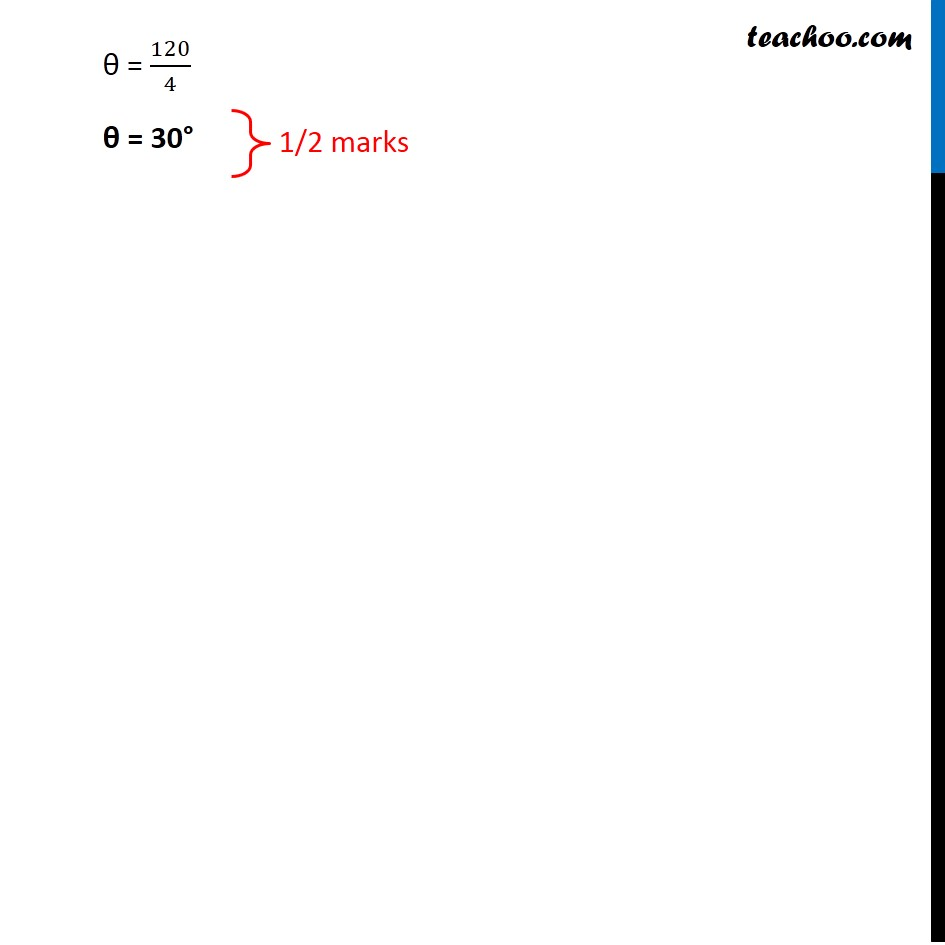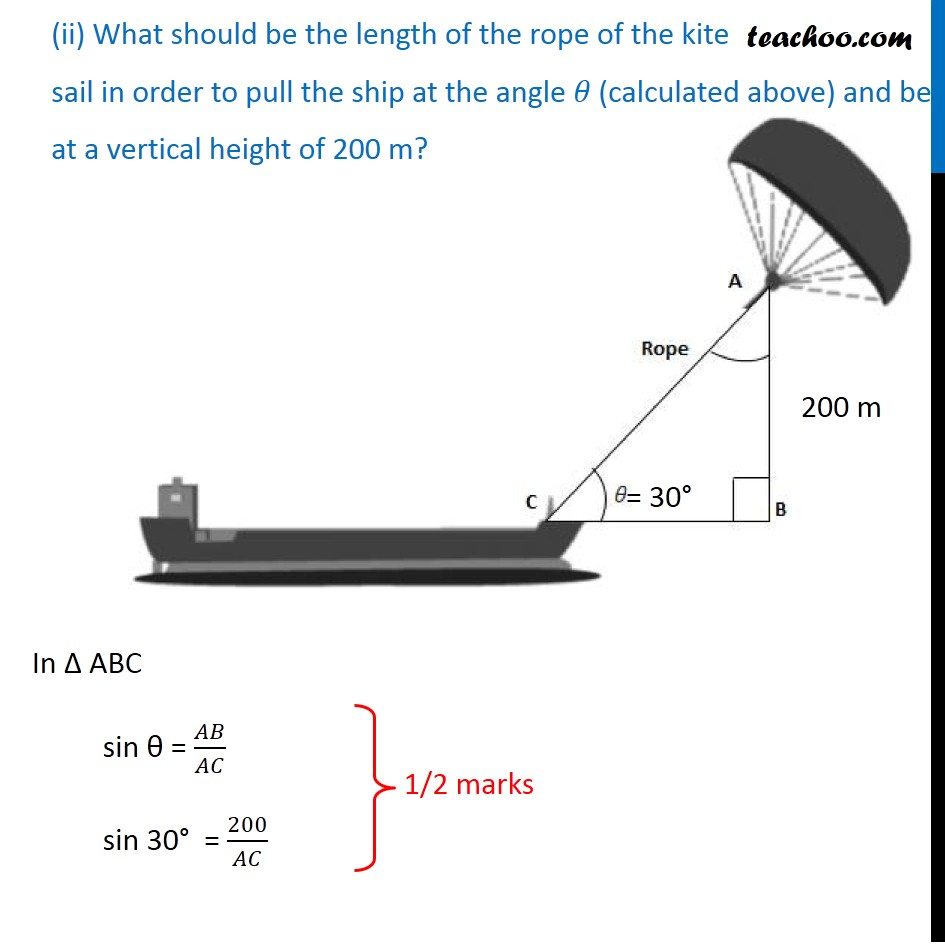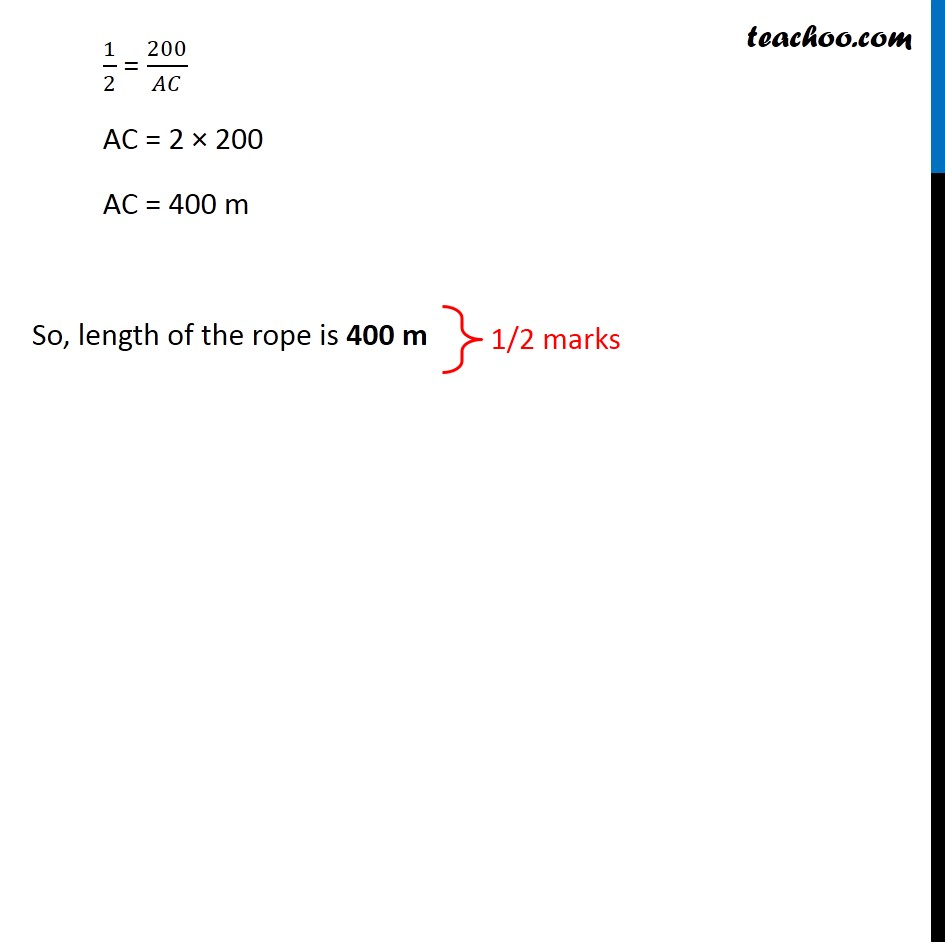Learn in your speed, with individual attention - Teachoo Maths 1-on-1 Class

### Transcript

Question 24 ‘Skysails’ is that genre of engineering science that uses extensive utilization of wind energy to move a vessel in the sea water. The ‘Skysails’ technology allows the towing kite to gain a height of anything between 100 metres – 300 metres. The sailing kite is made in such a way that it can be raised to its proper elevation and then brought back with the help of a ‘telescopic mast’ that enables the kite to be raised properly and effectively. Based on the following figure related to sky sailing, answer the questions: (i) In the given figure, if sin 𝜃 = cos (3𝜃 − 30°), where 𝜃 and 3𝜃 − 30° are acute angles, then find the value of 𝜃. Given sin 𝜃 = cos (3𝜃 − 30°) Writing sin θ = cos (90° – θ) cos (90° – 𝜃)= cos (3𝜃 − 30°) Comparing angles 90° – 𝜃 = 3𝜃 − 30° 90° + 30° = 3θ + θ 120° = 4θ 4θ = 120° θ = 120/4 θ = 30° (ii) What should be the length of the rope of the kite sail in order to pull the ship at the angle 𝜃 (calculated above) and be at a vertical height of 200 m? In Δ ABC sin θ = 𝐴𝐵/𝐴𝐶 sin 30° = 200/𝐴𝐶 1/2 = 200/𝐴𝐶 AC = 2 × 200 AC = 400 m So, length of the rope is 400 m